Equation Library

Equation Library

 Equation Library can be used to solve equations show vars,etc In this example we will use EQ LIB to solve a LINEAR MOTION formula.
 1-PressEQ LIB to access the EQUATION LIBRARY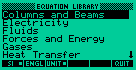Equation Library Application 2-Move the browser bar until MOTION press [ENTER] and go to Linear MotionLinear Motion 3-Press [VARS] to see the variable names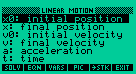Showing Units & Vars 4-Press [EQN] to see equations or formulas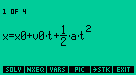Showing Formulas 5-Press [SOLV] in menu to start the solver modeStarting Solver 6-The VARS will appear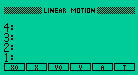VARS 7-Type a number and press the softkey closest to the unit to enter units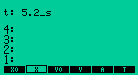Entering Units 8-Press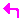and a softkey to see the result for the respective VAR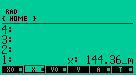Result

 Luck works better if you have faith. - Anonymous